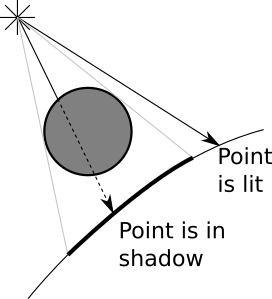# Shadows

Where there’s light and objects, there are shadows. So where are our shadows?

Let’s begin with a more fundamental question. Why should there be shadows? Shadows happen when there is a light, but its rays can’t reach an object because there’s some other object in the way.

You’ll notice that in the previous section we were worried with angles and vectors, but we only considered the light source and the point we wanted to paint, and completely ignored everything else happening in the scene - for example, an object getting in the way.

What we should do instead is to add a little bit of logic that says “if there’s an object between the point and the light, don’t add the illumination coming from this light”.

The two cases we want to distinguish are the following: //It turns out we already have all of the tools necessary to do this.

Let’s start with a directional light. We know $$P$$; that’s the point we’re interested in. We know $$\vec{L}$$; that’s part of the definition of the light. With $$P$$ and $$\vec{L}$$ we can define a ray, namely $$P + t\vec{L}$$, that goes from the point to the infinitely distant light source. Does this ray intersect any other object? If it doesn’t, there’s nothing between the point and the light, so we compute the illumination from this light and add it to the total. If it does, we ignore the light.

We already know how to compute the closest intersection between a ray and a sphere; we’re using it to trace the rays from the camera. We can reuse this to compute the closest intersection, if any, between the ray of light and the rest of the scene.

The parameters are slightly different, though. Instead of starting from the camera, rays start from $$P$$. The direction is not $$(V - O)$$ but $$\vec{L}$$. And we’re interested in intersections anywhere from next to $$P$$ to an infinite distance; this means $$t_{min} = 0$$ and $$t_{max} = +\infty$$.

We can treat point lights in a very similar way, with two exceptions. First, $$\vec{L}$$ is not given, but it’s very easy to compute it from the position of the light and $$P$$. Second, we’re interested in any intersection starting from $$P$$ but only up to $$L$$ (otherwise, objects beyond the light source could still create shadows!); so in this case $$t_{min} = 0$$ and $$t_{max} = 1$$.

There’s an interesting edge case we need to consider. Take the ray $$P + t\vec{L}$$. If we look for intersections starting from $$t_{min} = 0$$, we’ll most likely find $$P$$ itself at $$t = 0$$, since $$P$$ is indeed on a sphere, and $$P + 0\vec{L} = P$$; in other words, every object would be casting shadows over itself 1!

The simplest way to work around this is to use a small value $$\epsilon$$ instead of $$0$$ as the low end of the valid range for $$t$$. Geometrically, we’re saying we want the ray to start just a tiny bit off the surface where $$P$$ is, but not exactly at $$P$$. So the range will be $$[\epsilon, +\infty]$$ for directional lights, and $$[\epsilon, 1]$$ for point lights.

## Rendering with shadows

Let’s turn that into pseudocode.

In the previous version, TraceRay computes the closest ray-sphere intersection, and then computes lighting on the intersection. We need to extract the closest intersection code, since we want to reuse it to compute shadows:

ClosestIntersection(O, D, t_min, t_max) {
closest_t = inf
closest_sphere = NULL
for sphere in scene.Spheres {
t1, t2 = IntersectRaySphere(O, D, sphere)
if t1 in [t_min, t_max] and t1 < closest_t
closest_t = t1
closest_sphere = sphere
if t2 in [t_min, t_max] and t2 < closest_t
closest_t = t2
closest_sphere = sphere
}
return closest_sphere, closest_t
}

As a result TraceRay is much simpler:

TraceRay(O, D, t_min, t_max) {
closest_sphere, closest_t = ClosestIntersection(O, D, t_min, t_max)

if closest_sphere == NULL
return BACKGROUND_COLOR

P = O + closest_t*D  # Compute intersection
N = P - closest_sphere.center  # Compute sphere normal at intersection
N = N / length(N)
return closest_sphere.color*ComputeLighting(P, N, -D, sphere.specular)
}

Then we need to add the shadow check to ComputeLighting:

ComputeLighting(P, N, V, s) {
i = 0.0
for light in scene.Lights {
if light.type == ambient {
i += light.intensity
} else {
if light.type == point {
L = light.position - P
t_max = 1
} else {
L = light.direction
t_max = inf
}

# Shadow check
shadow_sphere, shadow_t = ClosestIntersection(P, L, 0.001, t_max)
if shadow_sphere != NULL
continue

# Diffuse
n_dot_l = dot(N, L)
if n_dot_l > 0
i += light.intensity*n_dot_l/(length(N)*length(L))

# Specular
if s != -1 {
R = 2*N*dot(N, L) - L
r_dot_v = dot(R, V)
if r_dot_v > 0
i += light.intensity*pow(r_dot_v/(length(R)*length(V)), s)
}
}
}
return i
}

Here’s what the freshly rendered scene looks like:

Source code and live demo >>

Now we’re getting somewhere.

Found an error? Everything is in Github.

1. More precisely, we want to avoid the situation where the point, not the whole object, casts shadow over itself; an object with a shape more complicated than a sphere (specifically, any concave object) can cast valid shadows over itself.